Statistical manifold

A manifoldendowed with a symmetric connection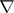and a Riemannian metric. This structure is abstracted from parametric statistics, i.e. inference from data distributed according to some unknown member of a parametrized family of probability distributions. The most cited such family is the multivariate normal distribution for data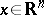, given bywith as parameters the mean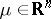and the covariance matrix. One thinks of the distributions themselves as points on a "surface" and the parameters as coordinates for these points. In this way any parametric family constitutes a manifoldwith allowable parametrizations providing admissible coordinate systems.

One can think of measures on a set as analogous to points in a plane and measurable functions (or random variables) as analogous to arrows which translate one point to another. The random variabletranslates the measureto anotherby the formula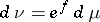, meaning thathas density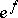with respect to. Composition of translation operations corresponds to adding the random variables (or arrows), and for pointsandthere is a unique translation movingto, provided one stays within an equivalence class of measures, subject to some regularity conditions. Such translation by a vector space of "arrows" is called an affine structure, and it is taken to be the essence of flat geometry.

Probability measures can be regarded as finite measures up to scale, since any finite (non-negative) measure can be uniquely scaled into one. In this sense, probability distributions live inside the flat geometry of an equivalence class as the finite measures. By choosing a finite number of linearly independent random variables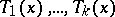, one obtains finite-dimensional affine subspaces of measures of the form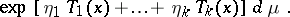For an open subset of the parameters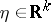, these measures are finite and can be scaled to probability measures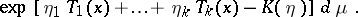They are the well-known exponential families. Their flatness can be related directly to their characterization in terms of sufficiency reduction.

General families of probability distributions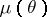are usually expressed as, whereis fixed. Geometrically this amounts to choosing an originand describing each distributionin terms of its displacement vector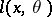from that origin. Differences in these displacement vectors give the displacement of one point in the family from another, and the derivatives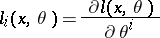give infinitesimal displacements or tangent vectors to the family, or manifold, at the point. The vector of random variables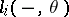is called the score and its components span the tangent space to the manifoldat.

By restrictingandto the span of the score components,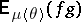defines an inner product on the tangent space at. Its matrix with respect to the score basis is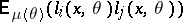, known as the Fisher information matrix. In this sense, the Fisher information defines an inner product on each tangent space of, i.e. a Riemannian metric. This is an observation going back to C.R. Rao, who noted that the multivariate normal family becomes a space of constant negative curvature under this metric [a7].

Because, asvaries, each score componentprovides a tangent vector at each point of, they are vector fields on(cf. Vector field). The second derivatives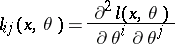give rates of change of these vector fields, but not intrinsically on, since these random variables will not generally lie in the span of the score components. By using the Fisher informationone can project the second derivatives onto the tangent spaces, thus defining intrinsically the rate of change of these vector fields on. Via linearity inand the Leibnitz rule inone defines, the rate of change of the vector fieldalong the vector field, for any two vector fieldsandon.is called the Amari-connection [a1]. S.-I. Amari noted that the dual connection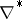with respect towas generally different from, i.e.is not the Riemannian or Levi-Civita connection of. One can therefore define a whole-parameter family of connections, so that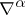and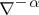are dual with respect to, and, in particular,is the Levi-Civita connection.

Amari showed that statistical divergences, such as Kullback–Leibler distances (cf. Kullback–Leibler-type distance measures), could be defined in terms of these structures. By duality, the rate of change of vector fields allows one to define the rate of change of-forms and hence of differentials of functions. For any function,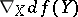is bilinear and symmetric inand. It therefore makes sense to try to realize the Fisher information in this form, i.e. to solve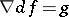[a6]. Solutions, if they exist, are uniquely determined by specifying the value ofat a single point. Unfortunatelymust be flat in order that a good supply of solutions exists. Let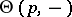be the solution whose differential vanishes at. Amari calls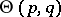the statistical divergence betweenand. For the-connection it is the Kullback–Leibler distance. It is easy to show that the minimum value of, forfixed andranging over a submanifold, occurs at the pointwhere the geodesic ofjoiningtomeetsorthogonally according to.

Much research on statistical manifolds centres on finding improved or more insightful asymptotic formulas. A connection is equivalent to specifying the rates of change of differentials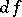, and the geometric form of second-order Taylor expansion. The "string (in statistics)strings" [a3] and "yoke (in statistics)yokes" [a5] of O.E. Barndorff-Nielsen and P. Blaesild (cf. also Yoke) define full geometric Taylor expansions in terms related to parametric statistics, seeking insight into results such as the Bartlett adjustment and the Barndorff-Nielsen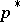formula [a4].